# Sampling

Currently, there are 9 functions associated with the sample verb in the sgsR package:

Algorithm Description Reference
sample_srs() Simple random
sample_systematic() Systematic
sample_strat() Stratified Queinnec, White, & Coops (2021)
sample_sys_strat() Systematic Stratified
sample_nc() Nearest centroid Melville & Stone (2016)
sample_clhs() Conditioned Latin hypercube Minasny & McBratney (2006)
sample_balanced() Balanced sampling Grafström, A. Lisic, J (2018)
sample_ahels() Adapted hypercube evaluation of a legacy sample Malone, Minasny, & Brungard (2019)
sample_existing() Sub-sampling an existing sample

## sample_srs

We have demonstrated a simple example of using the sample_srs() function in vignette("sgsR"). We will demonstrate additional examples below.

raster

The input required for sample_srs() is a raster. This means that sraster and mraster are supported for this function.

#--- perform simple random sampling ---#
sample_srs(
raster = sraster, # input sraster
nSamp = 200, # number of desired sample units
plot = TRUE
) # plot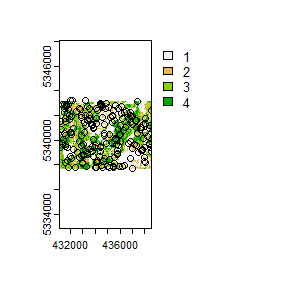#> Simple feature collection with 200 features and 0 fields
#> Geometry type: POINT
#> Dimension:     XY
#> Bounding box:  xmin: 431150 ymin: 5337750 xmax: 438550 ymax: 5343230
#> Projected CRS: UTM Zone 17, Northern Hemisphere
#> First 10 features:
#>                  geometry
#> 1  POINT (438350 5340950)
#> 2  POINT (434070 5341370)
#> 3  POINT (431370 5342930)
#> 4  POINT (434070 5342550)
#> 5  POINT (436230 5341730)
#> 6  POINT (431470 5340530)
#> 7  POINT (431910 5341990)
#> 8  POINT (435770 5338850)
#> 9  POINT (437430 5340550)
#> 10 POINT (434130 5341790)
sample_srs(
raster = mraster, # input mraster
nSamp = 200, # number of desired sample units
access = access, # define access road network
mindist = 200, # minimum distance sample units must be apart from one another
buff_inner = 50, # inner buffer - no sample units within this distance from road
buff_outer = 200, # outer buffer - no sample units further than this distance from road
plot = TRUE
) # plot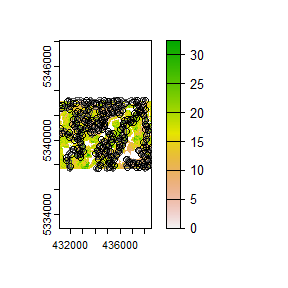#> Simple feature collection with 200 features and 0 fields
#> Geometry type: POINT
#> Dimension:     XY
#> Bounding box:  xmin: 431110 ymin: 5337730 xmax: 438530 ymax: 5343230
#> Projected CRS: UTM Zone 17, Northern Hemisphere
#> First 10 features:
#>                  geometry
#> 1  POINT (433150 5341490)
#> 2  POINT (432770 5339850)
#> 3  POINT (435030 5342170)
#> 4  POINT (435190 5339050)
#> 5  POINT (433890 5342970)
#> 6  POINT (437430 5343230)
#> 7  POINT (432830 5338610)
#> 8  POINT (435750 5338750)
#> 9  POINT (438110 5340390)
#> 10 POINT (434390 5341210)

## sample_systematic

The sample_systematic() function applies systematic sampling across an area with the cellsize parameter defining the resolution of the tessellation. The tessellation shape can be modified using the square parameter. Assigning TRUE (default) to the square parameter results in a regular grid and assigning FALSE results in a hexagonal grid.

The location of sample units can also be adjusted using the locations parameter, where centers takes the center, corners takes all corners, and random takes a random location within each tessellation. Random start points and translations are applied when the function is called.

#--- perform grid sampling ---#
sample_systematic(
raster = sraster, # input sraster
cellsize = 1000, # grid distance
plot = TRUE
) # plot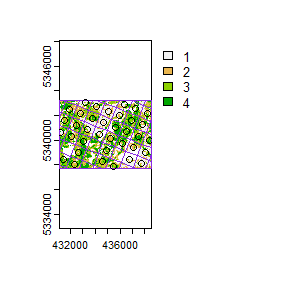#> Simple feature collection with 36 features and 0 fields
#> Geometry type: POINT
#> Dimension:     XY
#> Bounding box:  xmin: 431168.8 ymin: 5337896 xmax: 438419.4 ymax: 5343068
#> Projected CRS: UTM Zone 17, Northern Hemisphere
#> First 10 features:
#>                    geometry
#> 1  POINT (431888.7 5342495)
#> 2  POINT (431528.8 5341562)
#> 3  POINT (431168.8 5340629)
#> 4  POINT (433181.7 5343068)
#> 5  POINT (432821.7 5342135)
#> 6  POINT (432461.7 5341202)
#> 7  POINT (432101.7 5340269)
#> 8  POINT (431381.7 5338403)
#> 9  POINT (434114.7 5342708)
#> 10 POINT (433754.7 5341775)
#--- perform grid sampling ---#
sample_systematic(
raster = sraster, # input sraster
cellsize = 500, # grid distance
square = FALSE, # hexagonal tessellation
location = "random", # randomly sample within tessellation
plot = TRUE
) # plot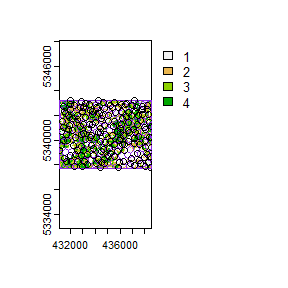#> Simple feature collection with 172 features and 0 fields
#> Geometry type: POINT
#> Dimension:     XY
#> Bounding box:  xmin: 431115.9 ymin: 5337766 xmax: 438548.6 ymax: 5343231
#> Projected CRS: UTM Zone 17, Northern Hemisphere
#> First 10 features:
#>                    geometry
#> 1  POINT (431408.7 5342764)
#> 2  POINT (432018.1 5343231)
#> 3  POINT (432010.1 5342921)
#> 4  POINT (431489.4 5342361)
#> 5  POINT (432320.7 5342849)
#> 6  POINT (431592.6 5342132)
#> 7  POINT (431988.8 5342504)
#> 8  POINT (432851.8 5343113)
#> 9  POINT (431344.6 5341478)
#> 10 POINT (431799.8 5342004)
sample_systematic(
raster = sraster, # input sraster
cellsize = 500, # grid distance
access = access, # define access road network
buff_outer = 200, # outer buffer - no sample units further than this distance from road
square = FALSE, # hexagonal tessellation
location = "corners", # take corners instead of centers
plot = TRUE
)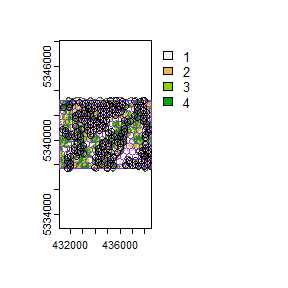#> Simple feature collection with 635 features and 0 fields
#> Geometry type: POINT
#> Dimension:     XY
#> Bounding box:  xmin: 431344.5 ymin: 5337707 xmax: 438536.1 ymax: 5343234
#> Projected CRS: UTM Zone 17, Northern Hemisphere
#> First 10 features:
#>                    geometry
#> 1  POINT (437944.1 5343234)
#> 2  POINT (437944.1 5343234)
#> 3  POINT (437688.5 5343100)
#> 4  POINT (437188.9 5343120)
#> 5  POINT (437688.5 5343100)
#> 6  POINT (437944.1 5343234)
#> 7  POINT (437920.8 5342657)
#> 8  POINT (437676.8 5342811)
#> 9  POINT (437688.5 5343100)
#> 10 POINT (437188.9 5343120)

## sample_strat

The sample_strat() contains two methods to perform sampling:

• "Queinnec" - Hierarchical sampling using a focal window to isolate contiguous groups of stratum pixels, which was originally developed by Martin Queinnec.

• "random" - Traditional stratified random sampling. This method ignores much of the functionality of the algorithm to allow users the capability to use standard stratified random sampling approaches without the use of a focal window to locate contiguous stratum cells.

### method = "Queinnec"

Queinnec, M., White, J. C., & Coops, N. C. (2021). Comparing airborne and spaceborne photon-counting LiDAR canopy structural estimates across different boreal forest types. Remote Sensing of Environment, 262(August 2020), 112510.

This algorithm uses moving window (wrow and wcol parameters) to filter the input sraster to prioritize sample unit allocation to where stratum pixels are spatially grouped, rather than dispersed individuals across the landscape.

Sampling is performed using 2 rules:

• Rule 1 - Sample within spatially grouped stratum pixels. Moving window defined by wrow and wcol.

• Rule 2 - If no additional sample units exist to satisfy desired sample size(nSamp), individual stratum pixels are sampled.

The rule applied to a select each sample unit is defined in the rule attribute of output samples. We give a few examples below:

#--- perform stratified sampling random sampling ---#
sample_strat(
sraster = sraster, # input sraster
nSamp = 200
) # desired sample size # plot
#> Simple feature collection with 200 features and 3 fields
#> Geometry type: POINT
#> Dimension:     XY
#> Bounding box:  xmin: 431110 ymin: 5337730 xmax: 438510 ymax: 5343210
#> Projected CRS: UTM Zone 17, Northern Hemisphere
#> First 10 features:
#>    strata type  rule               geometry
#> x       1  new rule1 POINT (433870 5343210)
#> x1      1  new rule2 POINT (436750 5338270)
#> x2      1  new rule2 POINT (437030 5339910)
#> x3      1  new rule2 POINT (434810 5341690)
#> x4      1  new rule2 POINT (435010 5341450)
#> x5      1  new rule2 POINT (435690 5342190)
#> x6      1  new rule2 POINT (432210 5342530)
#> x7      1  new rule2 POINT (438130 5337730)
#> x8      1  new rule2 POINT (434350 5341410)
#> x9      1  new rule2 POINT (433570 5342890)

In some cases, users might want to include an existing sample within the algorithm. In order to adjust the total number of sample units needed per stratum to reflect those already present in existing, we can use the intermediate function extract_strata().

This function uses the sraster and existing sample units and extracts the stratum for each. These sample units can be included within sample_strat(), which adjusts total sample units required per class based on representation in existing.

#--- extract strata values to existing samples ---#
e.sr <- extract_strata(
sraster = sraster, # input sraster
existing = existing
) # existing samples to add strata value to

TIP!

sample_strat() requires the sraster input to have an attribute named strata and will give an error if it doesn’t.

sample_strat(
sraster = sraster, # input sraster
nSamp = 200, # desired sample size
access = access, # define access road network
existing = e.sr, # existing sample with strata values
mindist = 200, # minimum distance sample units must be apart from one another
buff_inner = 50, # inner buffer - no sample units within this distance from road
buff_outer = 200, # outer buffer - no sample units further than this distance from road
plot = TRUE
) # plot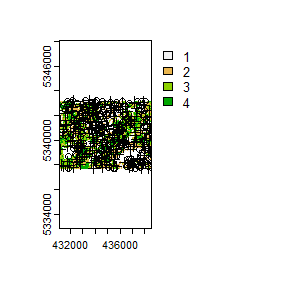#> Simple feature collection with 400 features and 3 fields
#> Geometry type: POINT
#> Dimension:     XY
#> Bounding box:  xmin: 431110 ymin: 5337710 xmax: 438550 ymax: 5343210
#> Projected CRS: UTM Zone 17, Northern Hemisphere
#> First 10 features:
#>    strata     type     rule               geometry
#> 1       1 existing existing POINT (433990 5340970)
#> 2       1 existing existing POINT (434070 5341430)
#> 3       1 existing existing POINT (434710 5340890)
#> 4       1 existing existing POINT (436770 5337790)
#> 5       1 existing existing POINT (436950 5338330)
#> 6       1 existing existing POINT (437670 5340770)
#> 7       1 existing existing POINT (433630 5341450)
#> 8       1 existing existing POINT (436510 5339830)
#> 9       1 existing existing POINT (437930 5343090)
#> 10      1 existing existing POINT (437970 5338770)

The code in the example above defined the mindist parameter, which specifies the minimum euclidean distance that new sample units must be apart from one another.

Notice that the sample units have type and rule attributes which outline whether they are existing or new, and whether rule1 or rule2 were used to select them. If type is existing (a user provided existing sample), rule will be existing as well as seen above.

sample_strat(
sraster = sraster, # input
nSamp = 200, # desired sample size
access = access, # define access road network
existing = e.sr, # existing samples with strata values
include = TRUE, # include existing sample in nSamp total
buff_outer = 200, # outer buffer - no samples further than this distance from road
plot = TRUE
) # plot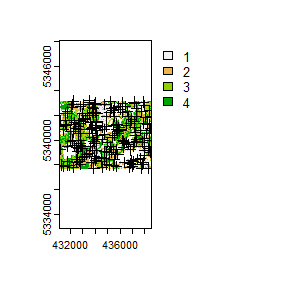#> Simple feature collection with 200 features and 3 fields
#> Geometry type: POINT
#> Dimension:     XY
#> Bounding box:  xmin: 431110 ymin: 5337710 xmax: 438550 ymax: 5343210
#> Projected CRS: UTM Zone 17, Northern Hemisphere
#> First 10 features:
#>    strata     type     rule               geometry
#> 1       1 existing existing POINT (433990 5340970)
#> 2       1 existing existing POINT (434070 5341430)
#> 3       1 existing existing POINT (434710 5340890)
#> 4       1 existing existing POINT (436770 5337790)
#> 5       1 existing existing POINT (436950 5338330)
#> 6       1 existing existing POINT (437670 5340770)
#> 7       1 existing existing POINT (433630 5341450)
#> 8       1 existing existing POINT (436510 5339830)
#> 9       1 existing existing POINT (437930 5343090)
#> 10      1 existing existing POINT (437970 5338770)

The include parameter determines whether existing sample units should be included in the total sample size defined by nSamp. By default, the include parameter is set as FALSE.

### method = "random

Stratified random sampling with equal probability for all cells (using default algorithm values for mindist and no use of access functionality). In essence this method perform the sample_srs algorithm for each stratum separately to meet the specified sample size.

#--- perform stratified sampling random sampling ---#
sample_strat(
sraster = sraster, # input sraster
method = "random", # stratified random sampling
nSamp = 200, # desired sample size
plot = TRUE
) # plot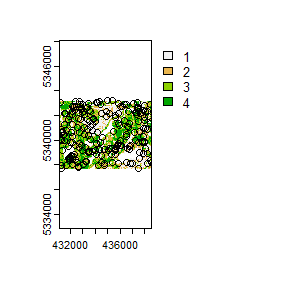#> Simple feature collection with 200 features and 1 field
#> Geometry type: POINT
#> Dimension:     XY
#> Bounding box:  xmin: 431150 ymin: 5337710 xmax: 438550 ymax: 5343230
#> Projected CRS: UTM Zone 17, Northern Hemisphere
#> First 10 features:
#>    strata               geometry
#> 1       1 POINT (438230 5338090)
#> 2       1 POINT (435170 5342170)
#> 3       1 POINT (432790 5342870)
#> 4       1 POINT (436610 5339290)
#> 5       1 POINT (432150 5342730)
#> 6       1 POINT (436490 5338230)
#> 7       1 POINT (436830 5338090)
#> 8       1 POINT (437570 5338530)
#> 9       1 POINT (438070 5340930)
#> 10      1 POINT (435330 5338810)

## sample_sys_strat

sample_sys_strat() function implements systematic stratified sampling on an sraster. This function uses the same functionality as sample_systematic() but takes an sraster as input and performs sampling on each stratum iteratively.

#--- perform grid sampling on each stratum separately ---#
sample_sys_strat(
sraster = sraster, # input sraster with 4 strata
cellsize = 1000, # grid size
plot = TRUE # plot output
)
#> Processing strata : 1
#> Processing strata : 2
#> Processing strata : 3
#> Processing strata : 4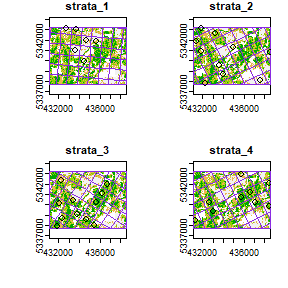#> Simple feature collection with 36 features and 1 field
#> Geometry type: POINT
#> Dimension:     XY
#> Bounding box:  xmin: 431295.5 ymin: 5337791 xmax: 438559.7 ymax: 5343166
#> Projected CRS: UTM Zone 17, Northern Hemisphere
#> First 10 features:
#>    strata                 geometry
#> 1       1 POINT (433756.3 5343014)
#> 2       1   POINT (432760 5343100)
#> 3       1 POINT (435663.2 5341846)
#> 4       1 POINT (434666.9 5341932)
#> 5       1 POINT (433584.8 5341022)
#> 6       1 POINT (434495.4 5339940)
#> 11      2 POINT (431900.6 5343166)
#> 21      2 POINT (433232.4 5342690)
#> 31      2 POINT (431424.9 5341834)
#> 41      2   POINT (431853 5340930)

Just like with sample_systematic() we can specify where we want our samples to fall within our tessellations. We specify location = "corners" below. Note that the tesselations are all saved to a list file when details = TRUE should the user want to save them.

sample_sys_strat(
sraster = sraster, # input sraster with 4 strata
cellsize = 500, # grid size
square = FALSE, # hexagon tessellation
location = "corners", # samples on tessellation corners
plot = TRUE # plot output
)
#> Processing strata : 1
#> Processing strata : 2
#> Processing strata : 3
#> Processing strata : 4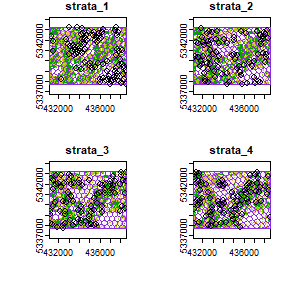#> Simple feature collection with 1178 features and 1 field
#> Geometry type: POINT
#> Dimension:     XY
#> Bounding box:  xmin: 431114.9 ymin: 5337707 xmax: 438555.3 ymax: 5343227
#> Projected CRS: UTM Zone 17, Northern Hemisphere
#> First 10 features:
#>    strata                 geometry
#> 1       1   POINT (438546 5337707)
#> 2       1   POINT (438546 5337707)
#> 3       1 POINT (438055.7 5337805)
#> 4       1   POINT (438546 5337707)
#> 5       1   POINT (438546 5337707)
#> 6       1 POINT (438385.7 5338181)
#> 7       1 POINT (438055.7 5337805)
#> 8       1 POINT (437838.8 5337996)
#> 9       1 POINT (438055.7 5337805)
#> 10      1 POINT (437018.5 5337718)

This sampling approach could be especially useful incombination with strat_poly() to ensure consistency of sampling accross specific management units.

#--- read polygon coverage ---#
poly <- system.file("extdata", "inventory_polygons.shp", package = "sgsR")
#> Reading layer inventory_polygons' from data source
#>   C:\Users\tgood\AppData\Local\Temp\RtmpWyvh0x\Rinst423c1f71584f\sgsR\extdata\inventory_polygons.shp'
#>   using driver ESRI Shapefile'
#> Simple feature collection with 632 features and 3 fields
#> Geometry type: MULTIPOLYGON
#> Dimension:     XY
#> Bounding box:  xmin: 431100 ymin: 5337700 xmax: 438560 ymax: 5343240
#> Projected CRS: UTM_Zone_17_Northern_Hemisphere

#--- stratify polygon coverage ---#
#--- specify polygon attribute to stratify ---#
attribute <- "NUTRIENTS"

#--- specify features within attribute & how they should be grouped ---#
#--- as a single vector ---#
features <- c("poor", "rich", "medium")

#--- get polygon stratification ---#
srasterpoly <- strat_poly(
poly = fri,
attribute = attribute,
features = features,
raster = sraster
)
#> Assigning a new crs. Use 'project' to transform a SpatRaster to a new crs

#--- systematatic stratified sampling for each stratum ---#
sample_sys_strat(
sraster = srasterpoly, # input sraster from strat_poly() with 3 strata
cellsize = 500, # grid size
square = FALSE, # hexagon tessellation
location = "random", # randomize plot location
plot = TRUE # plot output
)
#> Processing strata : 1
#> Processing strata : 2
#> Processing strata : 3
#> Simple feature collection with 178 features and 1 field
#> Geometry type: POINT
#> Dimension:     XY
#> Bounding box:  xmin: 431238.8 ymin: 5337701 xmax: 438551.3 ymax: 5343219
#> Projected CRS: UTM Zone 17, Northern Hemisphere
#> First 10 features:
#>    strata                 geometry
#> 1       1 POINT (438233.3 5342769)
#> 2       1 POINT (437807.8 5342666)
#> 3       1 POINT (435452.7 5342622)
#> 4       1 POINT (437927.9 5341893)
#> 5       1   POINT (434000 5342699)
#> 6       1   POINT (435029 5342649)
#> 7       1   POINT (435683 5342370)
#> 8       1 POINT (432709.2 5343172)
#> 9       1 POINT (433627.2 5342650)
#> 10      1 POINT (434414.8 5342760)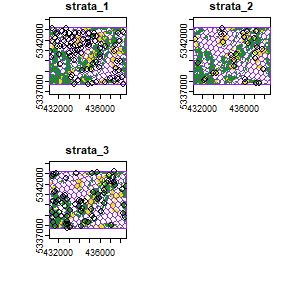## sample_nc

sample_nc() function implements the Nearest Centroid sampling algorithm described in Melville & Stone (2016). The algorithm uses kmeans clustering where the number of clusters (centroids) is equal to the desired sample size (nSamp).

Cluster centers are located, which then prompts the nearest neighbour mraster pixel for each cluster to be selected (assuming default k parameter). These nearest neighbours are the output sample units.

#--- perform simple random sampling ---#
sample_nc(
mraster = mraster, # input
nSamp = 25, # desired sample size
plot = TRUE
)
#> K-means being performed on 3 layers with 25 centers.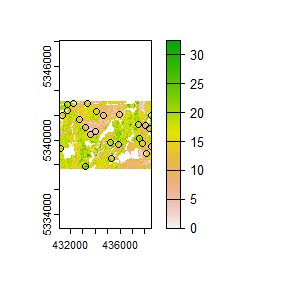#> Simple feature collection with 25 features and 4 fields
#> Geometry type: POINT
#> Dimension:     XY
#> Bounding box:  xmin: 431190 ymin: 5337910 xmax: 438530 ymax: 5342970
#> Projected CRS: UTM Zone 17, Northern Hemisphere
#> First 10 features:
#>        zq90 pzabove2  zsd kcenter               geometry
#> 43260 16.90     86.9 4.23       1 POINT (438390 5340930)
#> 57393 26.50     85.7 8.38       2 POINT (437570 5340170)
#> 23677  5.99     58.9 1.38       3 POINT (434650 5341970)
#> 39139  8.10     85.4 1.80       4 POINT (438030 5341150)
#> 52346  7.27     13.5 1.98       5 POINT (433610 5340430)
#> 99324 23.50     89.9 6.85       6 POINT (433210 5337910)
#> 22250  4.58     30.5 1.02       7 POINT (435950 5342050)
#> 63615 19.50     20.9 6.20       8 POINT (435190 5339830)
#> 5336  12.50     74.2 3.19       9 POINT (433370 5342950)
#> 80548 18.80     87.8 5.08      10 POINT (438150 5338930)

Altering the k parameter leads to a multiplicative increase in output sample units where total output samples = $$nSamp * k$$.

#--- perform simple random sampling ---#
samples <- sample_nc(
mraster = mraster, # input
k = 2, # number of nearest neighbours to take for each kmeans center
nSamp = 25, # desired sample size
plot = TRUE
)
#> K-means being performed on 3 layers with 25 centers.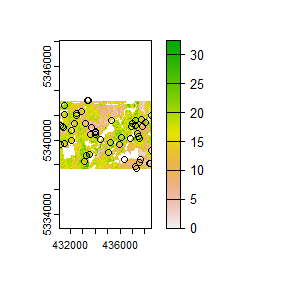#--- total samples = nSamp * k (25 * 2) = 50 ---#
nrow(samples)
#>  50

Visualizing what the kmeans centers and sample units looks like is possible when using details = TRUE. The $kplot output provides a quick visualization of where the centers are based on a scatter plot of the first 2 layers in mraster. Notice that the centers are well distributed in covariate space and chosen sample units are the closest pixels to each center (nearest neighbours). #--- perform simple random sampling with details ---# details <- sample_nc( mraster = mraster, # input nSamp = 25, # desired sample number details = TRUE ) #> K-means being performed on 3 layers with 25 centers. #--- plot ggplot output ---# details$kplot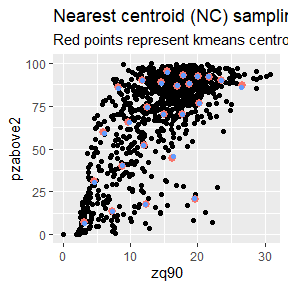## sample_clhs

sample_clhs() function implements conditioned Latin hypercube (clhs) sampling methodology from the clhs package.

TIP!

A number of other functions in the sgsR package help to provide guidance on clhs sampling including calculate_pop() and calculate_lhsOpt(). Check out these functions to better understand how sample numbers could be optimized.

The syntax for this function is similar to others shown above, although parameters like iter, which define the number of iterations within the Metropolis-Hastings process are important to consider. In these examples we use a low iter value for efficiency. Default values for iter within the clhs package are 10,000.

sample_clhs(
mraster = mraster, # input
nSamp = 200, # desired sample size
plot = TRUE, # plot
iter = 100
) # number of iterations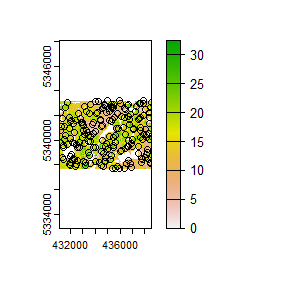The cost parameter defines the mraster covariate, which is used to constrain the clhs sampling. An example could be the distance a pixel is from road access (e.g. from calculate_distance() see example below), terrain slope, the output from calculate_coobs(), or many others.

#--- cost constrained examples ---#
#--- calculate distance to access layer for each pixel in mr ---#
mr.c <- calculate_distance(
raster = mraster, # input
access = access, # define access road network
plot = TRUE
) # plot
#>
|---------|---------|---------|---------|
=========================================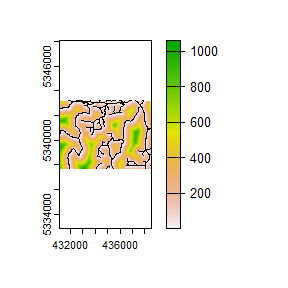sample_clhs(
mraster = mr.c, # input
nSamp = 250, # desired sample size
iter = 100, # number of iterations
cost = "dist2access", # cost parameter - name defined in calculate_distance()
plot = TRUE
) # plot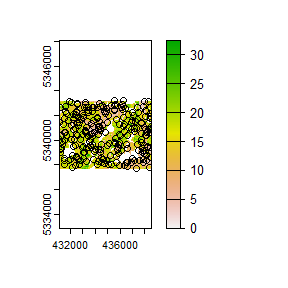## sample_balanced

The sample_balanced() algorithm performs a balanced sampling methodology from the stratifyR / SamplingBigData packages.

sample_balanced(
mraster = mraster, # input
nSamp = 200, # desired sample size
plot = TRUE
) # plot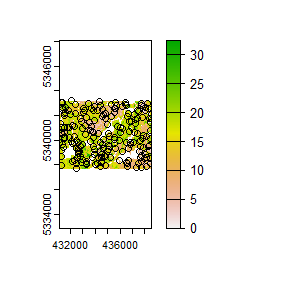#> Simple feature collection with 200 features and 0 fields
#> Geometry type: POINT
#> Dimension:     XY
#> Bounding box:  xmin: 431110 ymin: 5337710 xmax: 438550 ymax: 5343230
#> CRS:           +proj=utm +zone=17 +ellps=GRS80 +units=m +no_defs
#> First 10 features:
#>                  geometry
#> 1  POINT (432110 5343230)
#> 2  POINT (434370 5343150)
#> 3  POINT (431350 5343070)
#> 4  POINT (431290 5343050)
#> 5  POINT (435670 5343030)
#> 6  POINT (436470 5343030)
#> 7  POINT (438230 5342990)
#> 8  POINT (433470 5342970)
#> 9  POINT (437770 5342970)
#> 10 POINT (435270 5342870)
sample_balanced(
mraster = mraster, # input
nSamp = 100, # desired sample size
algorithm = "lcube", # algorithm type
access = access, # define access road network
buff_inner = 50, # inner buffer - no sample units within this distance from road
buff_outer = 200
) # outer buffer - no sample units further than this distance from road
#> Simple feature collection with 100 features and 0 fields
#> Geometry type: POINT
#> Dimension:     XY
#> Bounding box:  xmin: 431270 ymin: 5337790 xmax: 438410 ymax: 5343190
#> CRS:           +proj=utm +zone=17 +ellps=GRS80 +units=m +no_defs
#> First 10 features:
#>                  geometry
#> 1  POINT (434130 5339170)
#> 2  POINT (437110 5341490)
#> 3  POINT (434710 5341730)
#> 4  POINT (433030 5342950)
#> 5  POINT (434430 5338170)
#> 6  POINT (437270 5343030)
#> 7  POINT (435290 5342810)
#> 8  POINT (438170 5339970)
#> 9  POINT (438210 5337910)
#> 10 POINT (436130 5339090)

## sample_ahels

The sample_ahels() function performs the adapted Hypercube Evaluation of a Legacy Sample (ahels) algorithm usingexisting sample data and an mraster. New sample units are allocated based on quantile ratios between the existing sample and mraster covariate dataset.

This algorithm was adapted from that presented in the paper below, which we highly recommend.

Malone BP, Minansy B, Brungard C. 2019. Some methods to improve the utility of conditioned Latin hypercube sampling. PeerJ 7:e6451 DOI 10.7717/peerj.6451

This algorithm:

1. Determines the quantile distributions of existing sample units and mraster covariates.

2. Determines quantiles where there is a disparity between sample units and covariates.

3. Prioritizes sampling within those quantile to improve representation.

To use this function, user must first specify the number of quantiles (nQuant) followed by either the nSamp (total number of desired sample units to be added) or the threshold (sampling ratio vs. covariate coverage ratio for quantiles - default is 0.9) parameters.

#--- remove type variable from existing  - causes plotting issues ---#

existing <- existing %>% select(-type)

sample_ahels(
mraster = mraster,
existing = existing, # existing sample
plot = TRUE
) # plot
#> Simple feature collection with 298 features and 7 fields
#> Geometry type: POINT
#> Dimension:     XY
#> Bounding box:  xmin: 431110 ymin: 5337710 xmax: 438550 ymax: 5343210
#> Projected CRS: UTM Zone 17, Northern Hemisphere
#> First 10 features:
#>      type.x  zq90 pzabove2  zsd strata type.y  rule               geometry
#> 1  existing  4.20     11.7 0.89      1    new rule1 POINT (433990 5340970)
#> 2  existing  7.15     36.7 1.88      1    new rule2 POINT (434070 5341430)
#> 3  existing  2.82      2.9 0.44      1    new rule2 POINT (434710 5340890)
#> 4  existing  8.59     99.4 1.67      1    new rule2 POINT (436770 5337790)
#> 5  existing 10.50     89.9 2.54      1    new rule2 POINT (436950 5338330)
#> 6  existing 10.40     91.4 2.55      1    new rule2 POINT (437670 5340770)
#> 7  existing  3.56     15.4 0.68      1    new rule2 POINT (433630 5341450)
#> 8  existing  8.63     80.5 2.07      1    new rule2 POINT (436510 5339830)
#> 9  existing  3.05     11.9 0.61      1    new rule2 POINT (437930 5343090)
#> 10 existing 10.10     11.9 2.92      1    new rule2 POINT (437970 5338770)

TIP!

Notice that no threshold, nSamp, or nQuant were defined. That is because the default setting for threshold = 0.9 and nQuant = 10.

The first matrix output shows the quantile ratios between the sample and the covariates. A value of 1.0 indicates that the sample is representative of quantile coverage. Values > 1.0 indicate over representation of sample units, while < 1.0 indicate under representation.

sample_ahels(
mraster = mraster,
existing = existing, # existing sample
nQuant = 20, # define 20 quantiles
nSamp = 300
) # desired sample size
#> Simple feature collection with 500 features and 7 fields
#> Geometry type: POINT
#> Dimension:     XY
#> Bounding box:  xmin: 431110 ymin: 5337710 xmax: 438550 ymax: 5343230
#> Projected CRS: UTM Zone 17, Northern Hemisphere
#> First 10 features:
#>      type.x  zq90 pzabove2  zsd strata type.y  rule               geometry
#> 1  existing  4.20     11.7 0.89      1    new rule1 POINT (433990 5340970)
#> 2  existing  7.15     36.7 1.88      1    new rule2 POINT (434070 5341430)
#> 3  existing  2.82      2.9 0.44      1    new rule2 POINT (434710 5340890)
#> 4  existing  8.59     99.4 1.67      1    new rule2 POINT (436770 5337790)
#> 5  existing 10.50     89.9 2.54      1    new rule2 POINT (436950 5338330)
#> 6  existing 10.40     91.4 2.55      1    new rule2 POINT (437670 5340770)
#> 7  existing  3.56     15.4 0.68      1    new rule2 POINT (433630 5341450)
#> 8  existing  8.63     80.5 2.07      1    new rule2 POINT (436510 5339830)
#> 9  existing  3.05     11.9 0.61      1    new rule2 POINT (437930 5343090)
#> 10 existing 10.10     11.9 2.92      1    new rule2 POINT (437970 5338770)

Notice that the total number of samples is 500. This value is the sum of existing units (200) and number of sample units defined by nSamp = 300.

## sample_existing

Acknowledging that existing sample networks are common is important. There is significant investment into these samples, and in order to keep inventories up-to-date, we often need to collect new data for sample units. The sample_existing algorithm provides the user with methods for sub-sampling an existing sample network should the financial / logistical resources not be available to collect data at all sample units. The functions allows users to choose between algorithm types using (type = "clhs" - default, type = "balanced", type = "srs", type = "strat"). Differences in type result in calling internal sample_existing_*() functions (sample_existing_clhs() (default), sample_existing_balanced(), sample_existing_srs(), sample_existing_strat()). These functions are not exported to be used stand-alone, however they employ the same functionality as their sample_clhs() etc counterparts.

While using sample_existing(), should the user wish to specify algorithm specific parameters (e.g. algorithm = "lcube" in sample_balanced() or allocation = "equal" in sample_strat()), they can specify within sample_existing() as if calling the function directly.

I give applied examples for all methods below that are based on the following scenario:

• We have a systematic sample where sample units are 200m apart.

• We know we only have resources to sample 300 of them.

• We have some ALS data available (mraster), which we can use to improve knowledge of the metric populations.

See our existing sample for the scenario below.

#--- generate existing samples and extract metrics ---#
existing <- sample_systematic(raster = mraster, cellsize = 200, plot = TRUE)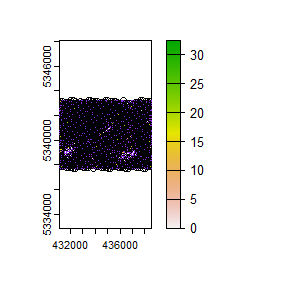#--- sub sample using ---#
e <- existing %>%
extract_metrics(mraster = mraster, existing = .)

### sample_existing(type = "clhs")

The algorithm is unique in that it has two fundamental approaches:

1. Sample exclusively using existing and the attributes it contains.
#--- sub sample using ---#
sample_existing(existing = e, nSamp = 300, type = "clhs")
#> Simple feature collection with 300 features and 3 fields
#> Geometry type: POINT
#> Dimension:     XY
#> Bounding box:  xmin: 431120.3 ymin: 5337710 xmax: 438559.5 ymax: 5343227
#> Projected CRS: UTM Zone 17, Northern Hemisphere
#> First 10 features:
#>      zq90 pzabove2  zsd                 geometry
#> 753 11.70     81.1 2.90 POINT (438051.7 5341757)
#> 471 15.20     88.4 4.10   POINT (436244 5340396)
#> 210 20.90     89.8 5.30 POINT (435228.4 5338924)
#> 299  2.73      7.9 0.43 POINT (437490.6 5339211)
#> 584 16.20     85.1 3.79 POINT (431998.8 5341803)
#> 500 17.90     83.6 4.88 POINT (435479.8 5340706)
#> 282 22.00     94.3 5.91 POINT (432935.7 5339853)
#> 79  22.90     95.7 5.59 POINT (432938.2 5338439)
#> 405 11.20     72.3 2.99 POINT (436584.2 5339944)
#> 551 21.60     78.1 6.77 POINT (431772.8 5341633)
1. Sub-sampling using raster distributions

Our systematic sample of ~900 plots is fairly comprehensive, however we can generate a true population distribution through the inclusion of the ALS metrics in the sampling process. The metrics will be included in internal latin hypercube sampling to help guide sub-sampling of existing.

#--- sub sample using ---#
sample_existing(
existing = existing, # our existing sample
nSamp = 300, # desired sample size
raster = mraster, # include mraster metrics to guide sampling of existing
plot = TRUE
) # plot
#> Simple feature collection with 300 features and 3 fields
#> Geometry type: POINT
#> Dimension:     XY
#> Bounding box:  xmin: 431120.3 ymin: 5337705 xmax: 438559.5 ymax: 5343227
#> CRS:           +proj=utm +zone=17 +ellps=GRS80 +units=m +no_defs
#> First 10 features:
#>      zq90 pzabove2  zsd                 geometry
#> 435 15.00     96.6 3.37 POINT (435621.9 5340282)
#> 627 13.50     93.6 3.32   POINT (433413 5341805)
#> 577 12.60     91.7 3.33 POINT (437714.1 5340795)
#> 137  2.54      5.8 0.39 POINT (438511.3 5337855)
#> 491 12.70     70.9 3.67 POINT (433103.3 5341041)
#> 562  4.76     54.6 1.09 POINT (433951.3 5341326)
#> 465  2.20      0.5 0.28 POINT (435055.7 5340564)
#> 806  9.01     19.9 2.57 POINT (434146.8 5342712)
#> 408 19.50     83.8 5.21 POINT (437178.3 5339861)
#> 555 16.30     88.0 3.81   POINT (432565 5341521)

The sample distribution again mimics the population distribution quite well! Now lets try using a cost variable to constrain the sub-sample.

#--- create distance from roads metric ---#
dist <- calculate_distance(raster = mraster, access = access)
#>
|---------|---------|---------|---------|
=========================================

#--- sub sample using ---#
sample_existing(
existing = existing, # our existing sample
nSamp = 300, # desired sample size
raster = dist, # include mraster metrics to guide sampling of existing
cost = 4, # either provide the index (band number) or the name of the cost layer
plot = TRUE
) # plot
#> Simple feature collection with 300 features and 4 fields
#> Geometry type: POINT
#> Dimension:     XY
#> Bounding box:  xmin: 431150.8 ymin: 5337710 xmax: 438539.2 ymax: 5343225
#> CRS:           +proj=utm +zone=17 +ellps=GRS80 +units=m +no_defs
#> First 10 features:
#>      zq90 pzabove2  zsd dist2access                 geometry
#> 148 12.20     37.4 2.78    10.70795 POINT (434380.4 5338639)
#> 650 22.80     92.6 5.85   172.47522   POINT (438364 5341107)
#> 709 16.70     89.6 3.94   366.27366 POINT (436241.4 5341811)
#> 853  3.86     17.3 0.77    37.49111 POINT (437937.5 5342379)
#> 743 12.30     97.4 2.69   141.04639 POINT (435873.3 5342064)
#> 130  6.91     78.7 1.51   102.78322   POINT (437125 5338050)
#> 868  5.42     40.1 1.26    72.23424   POINT (436183 5342829)
#> 312 17.90     90.4 3.80    49.56309 POINT (432567.5 5340107)
#> 872 22.90     98.1 4.08   145.69317 POINT (437371.3 5342661)
#> 198 19.30     93.5 4.42    73.33125 POINT (432851.9 5339259)

Finally, should the user wish to further constrain the sample based on access like other sampling approaches in sgsR that is also possible.

#--- ensure access and existing are in the same CRS ---#

sf::st_crs(existing) <- sf::st_crs(access)

#--- sub sample using ---#
sample_existing(
existing = existing, # our existing sample
nSamp = 300, # desired sample size
raster = dist, # include mraster metrics to guide sampling of existing
cost = 4, # either provide the index (band number) or the name of the cost layer
access = access, # roads layer
buff_inner = 50, # inner buffer - no sample units within this distance from road
buff_outer = 300, # outer buffer - no sample units further than this distance from road
plot = TRUE
) # plot
#> Simple feature collection with 300 features and 4 fields
#> Geometry type: POINT
#> Dimension:     XY
#> Bounding box:  xmin: 431120.3 ymin: 5337708 xmax: 438539.2 ymax: 5343227
#> CRS:           +proj=utm +zone=17 +ellps=GRS80 +units=m +no_defs
#> First 10 features:
#>      zq90 pzabove2  zsd dist2access                 geometry
#> 404 12.30     88.7 2.77   186.59599 POINT (437089.5 5342095)
#> 302  9.47     35.0 2.69    88.83060 POINT (434177.3 5341496)
#> 399  9.27     51.1 2.38   136.12306   POINT (434911 5342402)
#> 187  8.94     83.6 2.13   119.43920 POINT (437942.6 5339551)
#> 382 22.80     94.9 6.54    54.34220 POINT (437259.6 5341869)
#> 418 10.60     86.1 2.45    56.76280 POINT (434740.9 5342628)
#> 135 19.20     89.8 4.15   288.56785 POINT (432143.5 5339965)
#> 436  7.62     41.7 1.96   176.47194 POINT (435164.9 5342770)
#> 249 17.50     95.1 3.05    57.83165 POINT (432509.1 5341125)
#> 390 15.00     75.1 3.54   215.59635 POINT (432930.6 5342681)

TIP!

The greater constraints we add to sampling, the less likely we will have strong correlations between the population and sample, so its always important to understand these limitations and plan accordingly.

### sample_existing(type = "balanced")

When type = "balanced" users can define all parameters that are found within sample_balanced(). This means that one can change the algorithm, p etc.

sample_existing(existing = e, nSamp = 300, type = "balanced")
#> Simple feature collection with 300 features and 3 fields
#> Geometry type: POINT
#> Dimension:     XY
#> Bounding box:  xmin: 431150.8 ymin: 5337702 xmax: 438539.2 ymax: 5343227
#> Projected CRS: UTM Zone 17, Northern Hemisphere
#> First 10 features:
#>    zq90 pzabove2  zsd                 geometry
#> 6  11.8     71.7 3.31 POINT (432232.4 5337730)
#> 7  18.4     58.6 5.60 POINT (432430.5 5337702)
#> 11 18.0     48.6 4.87 POINT (431864.3 5337984)
#> 13 15.2     96.1 3.57 POINT (432260.3 5337928)
#> 15 12.6     83.5 3.19 POINT (432656.4 5337873)
#> 18 25.7     87.5 7.40 POINT (433250.5 5337789)
#> 19 15.0     95.6 3.50 POINT (433448.6 5337761)
#> 20 16.5     90.1 4.20 POINT (433844.7 5337705)
#> 23 17.9     93.5 4.08 POINT (431496.1 5338238)
#> 26 14.0     87.6 3.84 POINT (432090.2 5338154)
sample_existing(existing = e, nSamp = 300, type = "balanced", algorithm = "lcube")
#> Simple feature collection with 300 features and 3 fields
#> Geometry type: POINT
#> Dimension:     XY
#> Bounding box:  xmin: 431122.9 ymin: 5337708 xmax: 438536.7 ymax: 5343199
#> Projected CRS: UTM Zone 17, Northern Hemisphere
#> First 10 features:
#>      zq90 pzabove2  zsd                 geometry
#> 882 17.40     87.9 5.05 POINT (435418.8 5343138)
#> 700  9.32     55.4 2.54   POINT (434261 5342090)
#> 299  2.73      7.9 0.43 POINT (437490.6 5339211)
#> 373 14.80     92.4 3.57 POINT (437546.5 5339607)
#> 41  16.10     95.7 3.59 POINT (435258.9 5337708)
#> 126  9.23     86.8 2.12 POINT (436332.9 5338162)
#> 236 21.70     88.7 4.55 POINT (435850.5 5339038)
#> 524 18.50     62.3 4.86 POINT (432933.2 5341267)
#> 169 16.20     85.7 3.54   POINT (432824 5339061)
#> 735 10.60     56.9 2.70   POINT (434289 5342288)

### sample_existing(type = "srs")

The simplest, type = srs, randomly selects sample units.

sample_existing(existing = e, nSamp = 300, type = "srs")
#> Simple feature collection with 300 features and 3 fields
#> Geometry type: POINT
#> Dimension:     XY
#> Bounding box:  xmin: 431128 ymin: 5337708 xmax: 438536.7 ymax: 5343194
#> Projected CRS: UTM Zone 17, Northern Hemisphere
#> First 10 features:
#>      zq90 pzabove2  zsd                 geometry
#> 748 20.20     87.9 5.63 POINT (437061.5 5341897)
#> 664 18.30     92.7 3.88 POINT (434035.1 5341920)
#> 800  9.56     51.7 2.64 POINT (432760.5 5342907)
#> 638 21.70     84.4 5.01 POINT (435789.5 5341470)
#> 222 12.90     94.5 2.05 POINT (432681.8 5339485)
#> 367 14.80     94.3 2.65 POINT (436160.2 5339802)
#> 553 15.00     95.6 3.51 POINT (432168.9 5341577)
#> 61  16.00     68.6 3.91 POINT (434890.7 5337961)
#> 77  20.80     95.8 3.79 POINT (432542.2 5338495)
#> 174 17.10     33.8 5.66 POINT (434210.3 5338865)

### sample_existing(type = "strat")

When type = "strat", existing must have an attribute named strata (just like how sample_strat() requires a strata layer). If it doesnt exist you will get an error. Lets define an sraster so that we are compliant.

sraster <- strat_kmeans(mraster = mraster, nStrata = 4)

e_strata <- extract_strata(sraster = sraster, existing = e)

When we do have a strata attribute, the function works very much the same as sample_strat() in that is allows the user to define the allocation method ("prop" - defaults, "optim", "manual", "equal").

#--- proportional stratified sampling of existing ---#
sample_existing(existing = e_strata, nSamp = 300, type = "strat", allocation = "prop")
#> Simple feature collection with 301 features and 4 fields
#> Geometry type: POINT
#> Dimension:     XY
#> Bounding box:  xmin: 431100 ymin: 5337758 xmax: 438536.7 ymax: 5343217
#> Projected CRS: UTM Zone 17, Northern Hemisphere
#> First 10 features:
#>     strata zq90 pzabove2  zsd                 geometry
#> 150      1 19.6     95.4 4.13 POINT (434776.5 5338583)
#> 387      1 18.0     88.4 3.25 POINT (432821.4 5340475)
#> 825      1 18.8     87.9 4.56 POINT (438107.6 5342153)
#> 4        1 17.9     95.8 3.67 POINT (431836.3 5337786)
#> 577      1 12.6     91.7 3.33 POINT (437714.1 5340795)
#> 102      1 13.8     96.3 3.35 POINT (437691.2 5337768)
#> 330      1 13.1     92.1 3.41 POINT (436330.3 5339576)
#> 607      1 17.2     97.7 2.69 POINT (436949.8 5341105)
#> 854      1 14.6     89.6 3.30 POINT (438135.5 5342351)
#> 68       1 14.7     67.4 3.63   POINT (436277 5337766)

TIP!

Remember that when allocation = "equal", the nSamp value will be allocated for each strata.

We get 400 sample units in our output below because we have 4 strata and nSamp = 100.

#--- equal stratified sampling of existing ---#
sample_existing(existing = e_strata, nSamp = 100, type = "strat", allocation = "equal")
#> Simple feature collection with 400 features and 4 fields
#> Geometry type: POINT
#> Dimension:     XY
#> Bounding box:  xmin: 431100 ymin: 5337705 xmax: 438536.7 ymax: 5343222
#> Projected CRS: UTM Zone 17, Northern Hemisphere
#> First 10 features:
#>     strata zq90 pzabove2  zsd                 geometry
#> 227      1 15.9     94.9 3.63   POINT (433870 5339317)
#> 501      1 17.8     89.2 4.76 POINT (435677.8 5340678)
#> 509      1 16.3     71.2 4.65 POINT (437460.2 5340427)
#> 119      1 17.9     95.1 3.88 POINT (434748.5 5338385)
#> 4        1 17.9     95.8 3.67 POINT (431836.3 5337786)
#> 523      1 14.6     82.9 3.78 POINT (432735.1 5341295)
#> 790      1 17.7     79.0 4.70 POINT (438079.7 5341955)
#> 352      1 16.0     89.4 3.21 POINT (433189.6 5340221)
#> 621      1 17.2     90.4 3.56 POINT (432224.8 5341973)
#> 581      1 16.9     91.1 4.25 POINT (431404.7 5341887)
#--- manual stratified sampling of existing with user defined weights ---#
s <- sample_existing(existing = e_strata, nSamp = 100, type = "strat", allocation = "manual", weights = c(0.2, 0.6, 0.1, 0.1))

We can check the proportion of samples from each strata with:

#--- check proportions match weights ---#
table(s\$strata) / 100
#>
#>   1   2   3   4
#> 0.2 0.6 0.1 0.1

Finally, type = "optim allows for the user to define a raster metric to be used to optimize within strata variances.

#--- manual stratified sampling of existing with user defined weights ---#
sample_existing(existing = e_strata, nSamp = 100, type = "strat", allocation = "optim", raster = mraster, metric = "zq90")
#> Simple feature collection with 100 features and 4 fields
#> Geometry type: POINT
#> Dimension:     XY
#> Bounding box:  xmin: 431176.2 ymin: 5337705 xmax: 438534.1 ymax: 5343161
#> Projected CRS: UTM Zone 17, Northern Hemisphere
#> First 10 features:
#>     strata zq90 pzabove2  zsd                 geometry
#> 27       1 14.7     93.7 3.51 POINT (432288.3 5338126)
#> 319      1 21.3     98.5 4.00 POINT (434151.9 5339883)
#> 30       1 18.9     95.9 3.46 POINT (432882.4 5338043)
#> 532      1 14.1     98.1 3.38 POINT (434517.5 5341044)
#> 657      1 17.2     91.6 4.32 POINT (432450.7 5342143)
#> 577      1 12.6     91.7 3.33 POINT (437714.1 5340795)
#> 216      1 14.9     88.9 3.31 POINT (438397.1 5338477)
#> 704      1 16.4     91.5 4.37 POINT (435053.2 5341978)
#> 731      1 16.1     95.7 2.70 POINT (433496.8 5342400)
#> 100      1 15.6     89.7 4.10 POINT (437295.1 5337824)

We see from the output that we get 300 sample units that are a sub-sample of existing. The plotted output shows cumulative frequency distributions of the population (all existing` samples) and the sub-sample (the 300 samples we requested).